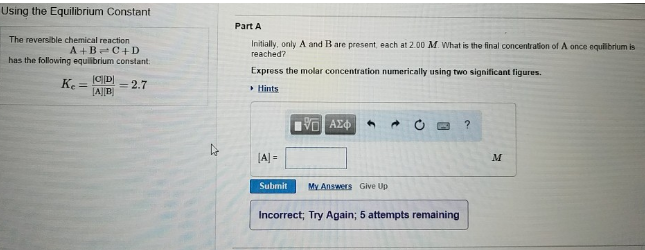# Problem: Using the Equilibrium constant The reversible chemical reaction A + B ⇌ C + D has the following equilibrium constant. Kc = [C][D]/[A][B] = 2.7 Part A Initially, only A and B are present, each at 2.00 M. What is the final concentration of A once equilibrium is reached?Express the molar concentration numerically using two significant figures.

###### FREE Expert Solution
85% (495 ratings)###### Problem Details

Using the Equilibrium constant

The reversible chemical reaction

A + B ⇌ C + D

has the following equilibrium constant.

Kc = [C][D]/[A][B] = 2.7

Part A

Initially, only A and B are present, each at 2.00 M. What is the final concentration of A once equilibrium is reached?

Express the molar concentration numerically using two significant figures.